1. /
2. CBSE
3. /
4. Class 07
5. /
6. Mathematics
7. /
8. NCERT Solutions for Class...

# NCERT Solutions for Class 7 Maths Exercise 11.2### myCBSEguide App

Download the app to get CBSE Sample Papers 2023-24, NCERT Solutions (Revised), Most Important Questions, Previous Year Question Bank, Mock Tests, and Detailed Notes.

NCERT solutions for Maths Perimeter and Area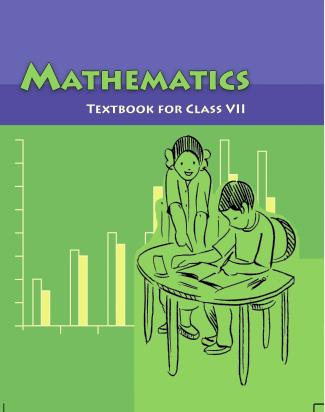## NCERT Solutions for Class 7 Maths Perimeter and Area

###### Question 1.Find the area of each of the following parallelograms: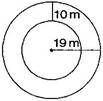We know that the area of parallelogram = base x height

(a) Here base = 7 cm and height = 4 cm

{tex}\therefore {/tex} Area of parallelogram = 7 x 4 = 28{tex}c{m^2}{/tex}

(b) Here base = 5 cm and height = 3 cm

{tex}\therefore {/tex} Area of parallelogram = 5 x 3 = 15 {tex}c{m^2}{/tex}

(c) Here base = 2.5 cm and height = 3.5 cm

{tex}\therefore {/tex} Area of parallelogram = 2.5 x 3.5 = 8.75 {tex}c{m^2}{/tex}

(d) Here base = 5 cm and height = 4.8 cm

{tex}\therefore {/tex} Area of parallelogram = 5 x 4.8 = 24 {tex}c{m^2}{/tex}

(e) Here base = 2 cm and height = 4.4 cm

{tex}\therefore {/tex} Area of parallelogram = 2 x 4.4 = 8.8 {tex}c{m^2}{/tex}

NCERT Solutions for Class 7 Maths Exercise 11.2

###### Question 2.Find the area of each of the following triangles: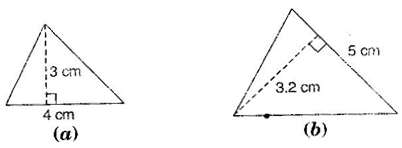We know that the area of triangle = {tex}\frac{1}{2}{/tex} x base x height

(a) Here, base = 4 cm and height = 3 cm

{tex}\therefore {/tex} Area of triangle = {tex}\frac{1}{2}{/tex} x 4 x 3 = 6 {tex}c{m^2}{/tex}

(b) Here, base = 5 cm and height = 3.2 cm

{tex}\therefore {/tex} Area of triangle = {tex}\frac{1}{2}{/tex} x 5 x 3.2 = 8 {tex}c{m^2}{/tex}

(c) Here, base = 3 cm and height = 4 cm

{tex}\therefore {/tex} Area of triangle = {tex}\frac{1}{2}{/tex} x 3 x 4 = 6{tex}c{m^2}{/tex}

(d) Here, base = 3 cm and height = 2 cm

{tex}\therefore {/tex} Area of triangle = {tex}\frac{1}{2}{/tex} x 3 x 2 = 3{tex}c{m^2}{/tex}

NCERT Solutions for Class 7 Maths Exercise 11.2

###### Question 3.Find the missing values:
 S. No. Base Hieght Area of the parallelogram a. 20 cm 246 {tex}c{m^2}{/tex} b. 15 cm 154.5{tex}c{m^2}{/tex} c. 84 cm 48.72 {tex}c{m^2}{/tex} d. 15.6 cm 16.38{tex}{m^2}{/tex}

We know that the area of parallelogram = base x height

(a) Here, base = 20 cm and area = 246 {tex}c{m^2}{/tex}

{tex}\therefore {/tex} Area of parallelogram = base x height

{tex} \Rightarrow {/tex} 246 = 20 x height {tex} \Rightarrow {/tex} height = {tex}\frac{{246}}{{20}}{/tex} = 12.3 cm

(b) Here, height = 15 cm and area = 154.5 cm2

{tex}\therefore {/tex} Area of parallelogram = base x height

{tex} \Rightarrow {/tex} 154.5 = base x 15 {tex} \Rightarrow {/tex} base = {tex}\frac{{154.5}}{{15}}{/tex} = 10.3 cm

(c) Here, height = 8.4 cm and area = 48.72{tex}c{m^2}{/tex}

{tex}\therefore {/tex} Area of parallelogram = base x height

{tex} \Rightarrow {/tex} 48.72 = base x 8.4 {tex} \Rightarrow {/tex} base = {tex}\frac{{48.72}}{{8.4}}{/tex} = 5.8 cm

(d) Here, base = 15.6 cm and area = 16.38 {tex}c{m^2}{/tex}

{tex}\therefore {/tex} Area of parallelogram = base x height

{tex} \Rightarrow {/tex} 16.38 = 15.6 x height {tex} \Rightarrow {/tex} height = {tex}\frac{{16.38}}{{15.6}}{/tex} = 1.05 cm

Thus, the missing values are:

 S. No. Base Hieght Area of the parallelogram a. 20 cm 12.3 cm 246 {tex}c{m^2}{/tex} b. 10.3 cm 15 cm 154.5{tex}c{m^2}{/tex} c. 5.8 cm 84 cm 48.72{tex}c{m^2}{/tex} d. 15.6 cm 1.05 16.38 {tex}c{m^2}{/tex}

NCERT Solutions for Class 7 Maths Exercise 11.2

###### Question 4.Find the missing values:
 Base Hieght Area of triangle 15 cm — 87 {tex}c{m^2}{/tex} — 31.4 mm 1256 {tex}m{m^2}{/tex} 22 cm — 170.5{tex}c{m^2}{/tex}

We know that the area of triangle = {tex}\frac{1}{2}{/tex} x base x height

In first row, base = 15 cm and area = 87 {tex}c{m^2}{/tex}

{tex}\therefore {/tex} 87 = {tex}\frac{1}{2}{/tex} x 15 x height {tex} \Rightarrow {/tex} height = {tex}\frac{{87 \times 2}}{{15}}{/tex} 11.6 cm

In second row, height = 31.4 mm and area = 1256 {tex}m{m^2}{/tex}

{tex}\therefore {/tex} 1256 = {tex}\frac{1}{2}{/tex} x base x 31.4 {tex} \Rightarrow {/tex} base = {tex}\frac{{1256 \times 2}}{{31.4}}{/tex} 80 mm

In third row, base = 22 cm and area = 170.5 {tex}c{m^2}{/tex}

{tex}\therefore {/tex} 170.5 = {tex}\frac{1}{2}{/tex} x 22 x height {tex} \Rightarrow {/tex} height = {tex}\frac{{170.5 \times 2}}{{22}}{/tex} 15.5 cm

Thus, the missing values are:

 Base Hieght Area of triangle 15 cm 11.6 cm 87 {tex}c{m^2}{/tex} 80 mm 31.4 mm 1256 {tex}m{m^2}{/tex} 22 cm 15.5 cm 170.5 {tex}c{m^2}{/tex}

NCERT Solutions for Class 7 Maths Exercise 11.2

###### Question 5.PQRS is a parallelogram. QM is the height from Q to SR and QN is the height from Q to PS. If SR = 12 cm and QM = 7.6 cm. Find:

(a) the area of the parallelogram PRS

(b) QN, if PS = 8 cm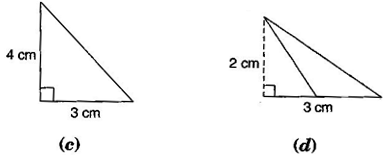Given: SR = 12 cm, QM= 7.6 cm, PS = 8 cm.

(a) Area of parallelogram = base x height

= 12 x 7.6 = 91.2 {tex}c{m^2}{/tex}

(b) Area of parallelogram = base x height

{tex} \Rightarrow {/tex} 91.2 = 8 x QN {tex} \Rightarrow {/tex} QN = {tex}\frac{{91.2}}{8}{/tex} = 11.4 cm

Question 6.DL and BM are the heights on sides AB and AD respectively of parallelogram ABCD. If the area of the parallelogram is 1470{tex}c{m^2}{/tex}, AB = 35 cm and AD = 49 cm, find the length of BM and DL.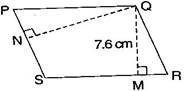Given: Area of parallelogram = 1470{tex}c{m^2}{/tex}

Base (AB) = 35 cm and base (AD) = 49 cm

Since Area of parallelogram = base x height

{tex} \Rightarrow {/tex} 1470 = 35 x DL {tex} \Rightarrow {/tex} DL = {tex}\frac{{1470}}{{35}}{/tex}

{tex} \Rightarrow {/tex} DL = 42 cm

Again, Area of parallelogram = base x height

{tex} \Rightarrow {/tex} 1470 = 49 x BM {tex} \Rightarrow {/tex} BM = {tex}\frac{{1470}}{{49}}{/tex}

{tex} \Rightarrow {/tex} BM = 30 cm

Thus, the lengths of DL and BM are 42 cm and 30 cm respectively.

NCERT Solutions for Class 7 Maths Exercise 11.2

###### Question 7.{tex}\Delta {/tex}ABC is right angled at A. AD is perpendicular to BC. If AB = 5 cm, BC = 13 cm and AC = 12 cm, find the area of {tex}\Delta {/tex}ABC. Also, find the length of AD.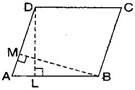In right angles triangle BAC, AB = 5 cm and AC = 12 cm

Area of triangle = {tex}\frac{1}{2}{/tex} x base x height = {tex}\frac{1}{2}{/tex} x AB x AC

= {tex}\frac{1}{2}{/tex} x 5 x 12 = 30{tex}c{m^2}{/tex}

Now, in {tex}\Delta {/tex}ABC,

Area of triangle ABC = {tex}\frac{1}{2}{/tex} x BC x AD

{tex} \Rightarrow {/tex} 30 = {tex}\frac{1}{2}{/tex} x 13 x AD {tex} \Rightarrow {/tex} AD = {tex}\frac{{30 \times 2}}{{13}}{/tex} = {tex}\frac{{60}}{{13}}{/tex} cm

NCERT Solutions for Class 7 Maths Exercise 11.2

###### Question 8.{tex}\Delta {/tex}ABC is isosceles with AB = AC = 7.5 cm and BC = 9 cm. The height AD from A to BC, is 6 cm. Find the area of {tex}\Delta {/tex}ABC. What will be the height from C to AB i.e., CE?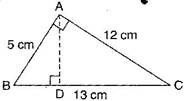In {tex}\Delta {/tex}ABC, AD = 6 cm and BC = 9 cm

Area of triangle = {tex}\frac{1}{2}{/tex} x base x height = {tex}\frac{1}{2}{/tex} x BC x AD

= {tex}\frac{1}{2}{/tex} x 9 x 6 = 27{tex}c{m^2}{/tex}

Again, Area of triangle = {tex}\frac{1}{2}{/tex} x base x height = {tex}\frac{1}{2}{/tex} x AB x CE

{tex} \Rightarrow {/tex} 27 = {tex}\frac{1}{2}{/tex} x 7.5 x CE {tex} \Rightarrow {/tex} CE = {tex}\frac{{27 \times 2}}{{7.5}}{/tex}

{tex} \Rightarrow {/tex} CE = 7.2 cm

Thus, height from C to AB i.e., CE is 7.2 cm.

## NCERT Solutions for Class 7 Maths Exercise 11.2

NCERT Solutions Class 7 Maths PDF (Download) Free from myCBSEguide app and myCBSEguide website. Ncert solution class 7 Maths includes text book solutions from Class 7 Maths Book . NCERT Solutions for CBSE Class 7 Maths have total 15 chapters. 7 Maths NCERT Solutions in PDF for free Download on our website. Ncert Maths class 7 solutions PDF and Maths ncert class 7 PDF solutions with latest modifications and as per the latest CBSE syllabus are only available in myCBSEguide.

## CBSE app for Students

To download NCERT Solutions for Class 7 Maths, Social Science Computer Science, Home Science, Hindi English, Maths Science do check myCBSEguide app or website. myCBSEguide provides sample papers with solution, test papers for chapter-wise practice, NCERT solutions, NCERT Exemplar solutions, quick revision notes for ready reference, CBSE guess papers and CBSE important question papers. Sample Paper all are made available through the best app for CBSE students and myCBSEguide website.Test Generator

Create question paper PDF and online tests with your own name & logo in minutes.myCBSEguide

Question Bank, Mock Tests, Exam Papers, NCERT Solutions, Sample Papers, Notes

### 8 thoughts on “NCERT Solutions for Class 7 Maths Exercise 11.2”

1. Excellent

2. Superb solution

3. Excellent … Work …

4. Superb…

5. It was very nice????????

6. Outstanding ????????

7. Outstanding ????????

8. It is very usefully to every student